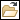Surfacing > Scan Tools > Importing Raw Data > To Import High Density Data
To Import High Density Data
1. Click Geometry >Sample Data from File. The Import Raw dialog box opens.
2. Click High Density.
3. Select a coordinate system. The Open dialog box opens.
4. Select a data file that you want to import. You can import a .igs (point-formatted), .ibl, .vda, or .pts file. The Raw Data dialog box opens.
5. Set the percentage of visible scan points by typing a value in the Visible Points Percent box.
6. Specify the direction for filtering raw data:
Scan Curves—Filters out raw data along scan curves.
Auto Direction Curves—Scan Tools determines an optimal direction and generates a set of curves from the original raw data.
Sections—Intersects the point cloud with sections to create raw data sets.
7. If you selected Scan Curves, define point spacing and section spacing as follows:
In the Curve Distance box, type a value for the distance between scan curves.
In the Points Tolerance box, type a value for the distance between points.
8. If you selected Auto Direction Curves, define point spacing and section spacing as follows:
In the Number of Sections box, type the number of sections to use to intersect a point cloud.
In the Proximity Zone box, type a value for the width of the zone that goes along the length of a section. Scan Tools processes data points that lie within this zone to create scan curves. The proximity zone should not be more than half the distance between two adjacent curves.
In the Points Tolerance box, type a value for the distance between points.
9. If you selected Sections, define the Section Type.
10. Define the Point/Section Spacing as follows:
In the Proximity Zone box, type the width of the zone that goes along the length of a section. Scan Tools processes data points that lie within that zone to create scan curves.
In the Points Tolerance box, type a value for the distance between points.
11. Click Preview or OK.